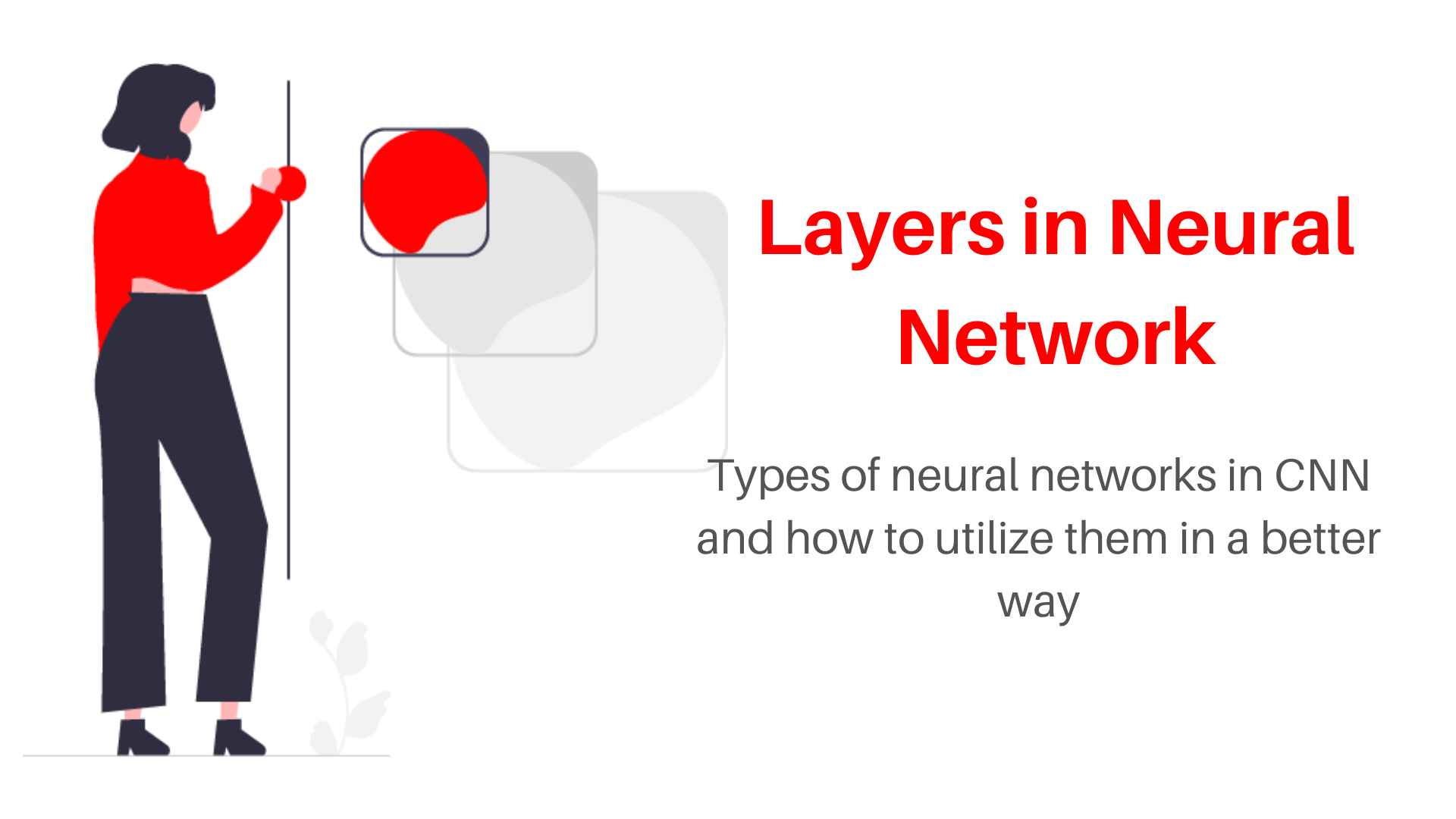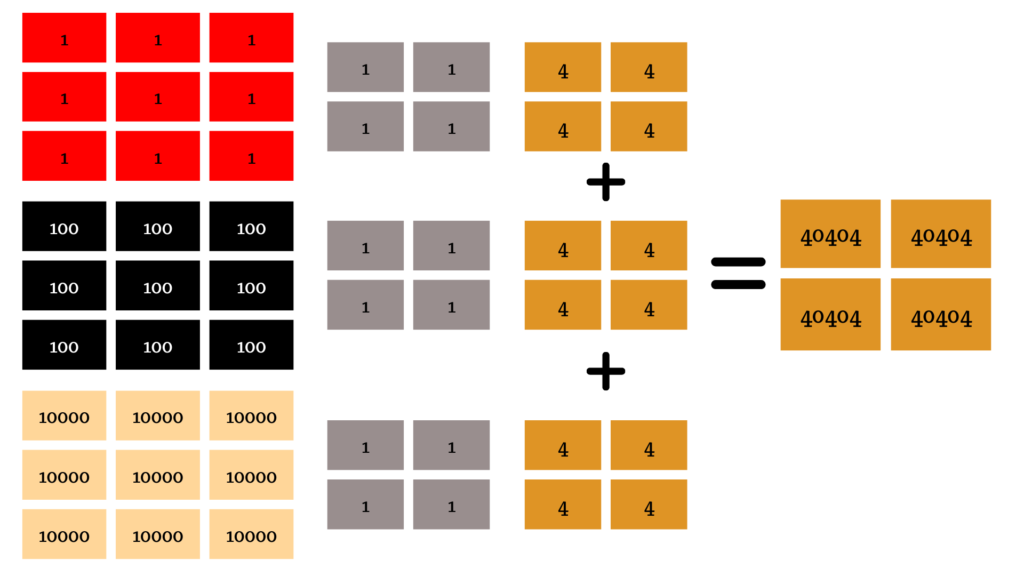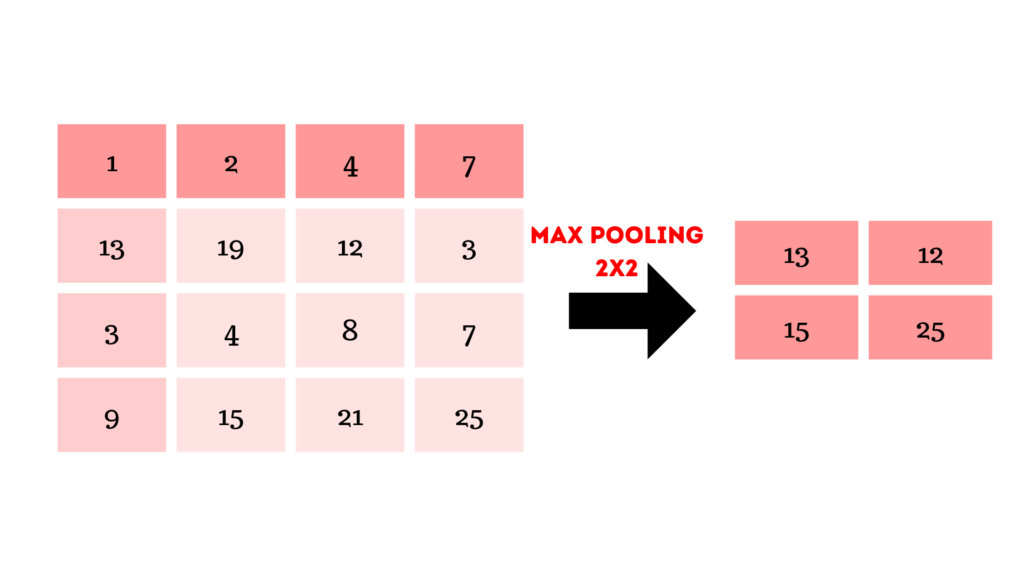# What are the layers in CNN: How to utilize them in a better way?## Introduction

Implementing a project on Image Segmentation, but lacking the fundamentals to building architecture and how layers in CNN are involved in it?

Understanding of Convolutional Neur...
Understanding of Convolutional Neural Network (CNN) — Deep Learning

In this blog, we explain the layers in CNN in terms of their different types, utilization, benefits! Layers in CNN(Convolutional Neural networks) are building blocks that are concatenated by individual layers to perform different tasks like Image recognition, object detection. It is very easy to understand, let’s get started.

## OverView

1. What is CNN and its architecture
2. Different Types of layers in CNN
1. Input layer
2. Convolutional layer
3. Stride and Padding
4. Pooling Layer
5. Fully Connected Layer in CNN
6. Dropout layer
7. Activation Function
8. Output layer
3. Implementation of layers in CNN using keras
4. Conclusion

## What is CNN and its architecture?

Convolutional neural networks are made up of an input layer, an output layer, and many hidden layers. Unlike in a typical neural network, the neurons in the layers of a convolutional network are arranged in 3-D(width, height, and convolutional neural networks are made up of an input layer, an output layer, and many hidden layers.

This allows the CNN to turn a three-dimensional input volume into a two-dimensional(2-D) output volume. The hidden layers are made up of convolution, pooling, normalizing, and fully connected layers. CNN’s use many convolution layers to filter input volumes to higher levels of abstraction.

Convolutional neural networks resemble feed-forward neural networks in appearance. CNN’s vary in that they explicitly presume that the inputs are images, allowing us to embed specific attributes in the architecture to identify specific patterns in the images. The spatial feature of the data is used by CNN. It means that CNNs perceive objects similar to how we observe various objects in nature.

We detect different items based on their shapes, sizes, and color. For eg, Those objects are made up of a variety of edges, corners, hue patches, and other elements. CNN may investigate images using detectors like edge detectors, corner detectors.

## Different types of layers in CNN

### Input layer in CNN

Input layer In CNN represents the pixel of the image in the form of a matrix.

Image data should be present in CNN’s input layer. As previously stated, image data is represented by a three-dimensional matrix. It needs to be reshaped into a single column. If you have an image with dimensions of 32x 32 =1024, you must convert it to 1024 x 1 before feeding it into the input. If you have “k” training examples, the input dimension will be (1024, k).

### Convolution layer in CNN

The convolutional layer uses a filter to apply a convolution operator to the input data and provides a feature map as an output. The convolution technique is used to extract high-level characteristics from the input image, such as edges. The first ConvLayer is used to collect low-level information including edges, color, and orientation. Adding more layers allows the architecture to adapt to high-level features as well, resulting in a network that understands all of the photos in the dataset. By sliding the filter over the input, we perform a convolution. Element-wise matrix multiplication is done at each position, and the result is added to the feature map

###### RGB input image (form of a matrix) applying a single filter using conv2D

If the input image size is NXN and the Filter size is KXK then the result of the convolution layer (feature map) will be given by using this formula (N x N) * (K x K) = (N-K +1) X (N – K + 1)

In the below, an input image is in form of a matrix where input image size N = 3, filter size k =2 and then output feature -> (3*3) *(2*2) = (3-2+1)*(3-2+1) = 2X2 .RGB input image with filter 2X2 and output feature 2×2

### Stride and Padding

#### strides

Stride is a component of convolutional neural networks, where neural networks are optimized for image and video data compression. It is a neural network filter parameter, that modifies the amount of movement over the image or video.

The below image explains how stride works, the number of pixels shifted across the input matrix is referred to as the stride. When the stride is 1, the filters are moved one pixel at a time. When the stride is 2, the filters are moved two pixels at a time, and so on. The diagram below shows how convolution would work with a stride of 2. Stride determines how the filter condenses around the input volume.

Padding effectively expands the area of an image that CNN processes, where the filter moves across the image scans and compresses each pixel. Padding is added to the image’s outer frame to allow for more space for the filter to cover in the image, allowing the kernel to work with image processing.

Padding is used to keep an image’s original size when applying a convolutional filter. It works by increasing the size of the area over which a convolutional neural network processes an image. The kernel is the neural network filter that moves across the image, scanning each pixel and converting the data into a smaller, or occasionally larger, format. When the filter does not fit the input image perfectly then we use padding. The addition of empty pixels around the edges of an image is referred to as padding.

Padding is used to avoid the major problems :

1. While performing the convolution operations, the final image will become small due to image shrinks with every operation.
2. While we slide the full kernels over the edge pixels, we may lose data at edges. So that we cannot perform full convolutions.

Padding the input image with additional empty pixels around the edges.

## Pooling layer in CNN

The pooling Layer in CNN is usually applied after a Convolutional Layer, this layer’s major goal is to lower the size of the convolved feature map to reduce computational expenses. This is accomplished by reducing the connections between layers and operating independently on each feature map. There are numerous sorts of Pooling operations, depending on the mechanism utilized.

The feature mapping dimensions are reduced by using pooling layers. So, the number of parameters to learn and the amount of processing in the network are both reduced.

The features contained in a region of the feature map generated by a convolution layer are summed up by the pooling layer. Rather than precisely positioned features created by the convolution layer, the following actions are conducted on aggregated features. As a result, the model is more resistant to changes in the position of features in the input image.

The largest element is obtained from the feature map in Max Pooling. The average of the elements in a predefined sized Image segment is calculated using Average Pooling. Sum Pooling calculates the total sum of the components in the predefined section. The Pooling Layer is typically used to connect the Convolutional Layer and the Fully-Connected Layer.

### Max pooling in CNN

Pooling that selects the maximum element from the region of the feature map covered by the filter is known as max pooling. So, the output of the max-pooling layer would be a feature map with the most prominent features from the previous feature map.

###### Max pooling Implementation using python
```import numpy as np
from keras.models import Sequential
from keras.layers import MaxPooling2D

"""define input image in form of matrix"""
image = np.array([[10,12,8,7],
[4,11,5,9],
[18,13,7,7],
[3,15,2,2]])
image = image.reshape(1, 4, 4, 1)

"""define model containing just a single max pooling layer"""
model = Sequential(
[MaxPooling2D(pool_size = 2, strides = 2)])

'''output prediction'''
output = model.predict(image)

'''print output image matrix after applying maxpooling'''
output = np.squeeze(output)
print(output)

```Max pooling with filter 2X2 and stride by 2

From the above image we can understand as follows:

Max(1,2,13,10) = 13, Max(4,7,12,3) = 12 , Max(3,4,11,15) = 15 ,Max(8,9,21,25) = 25

### Average pooling

The average of the items present in the region of the feature map covered by the filter is computed using average pooling.

Average pooling using Python

```import tensorflow
import numpy as np
from keras.models import Sequential
from keras.layers import AveragePooling2D

"""define input image in form of matrix"""
image = np.array([[10,12,8,7],
[4,11,5,9],
[18,13,7,7],
[3,15,2,2]])
image = image.reshape(1, 4, 4, 1)

"""define model containing just a single Average pooling layer"""
model = Sequential(
[AveargePooling2D(pool_size = 2, strides = 2)])

'''output prediction'''
output = model.predict(image)

'''print output image matrix after applying Average pooling layer'''
output = np.squeeze(output)
print(output)
```

In the image the above image, we get a feature map using a filter 2X2 with stride 2X2.

From the above image, We can understand like:

Avg(10,12,4,11) = 9.25 , Avg(6,7,5,9)= 6.75 , Avg(18,13,3,15)= 12.25, Avg(7,7,2,3) = 4.75

### Global pooling

Global Average Pooling is a pooling operation that is intended to replace fully connected layers in traditional. Rather than layering fully connected layers on top of the feature maps, we take the average of each feature map and feed the resulting vector directly into the activation layer.

Implementation of Global pooling using Keras

```import numpy as np
from keras.models import Sequential
from keras.layers import GlobalMaxPooling2D,GlobalAveragePooling2D

'''input-image in form of matrix'''
image = np.array([[10,12,8,7],
[4,11,5,9],
[18,13,7,7],
[3,15,2,2]])
image = image.reshape(1, 4, 4, 1)

'''define gmax_model containing just a single global-max pooling layer'''
gmax_model = Sequential(
[GlobalMaxPooling2D()])

'''define gavg_model containing just a single global-average pooling layer'''
gavg_model = Sequential(
[GlobalAveragePooling2D()])

'''output prediction'''
gmax_output = gmax_model.predict(image)
gavg_output = gavg_model.predict(image)

'''output image in form of matrix'''
gmax_output = np.squeeze(gmax_output)
gavg_output = np.squeeze(gavg_output)
print("gmax_output: ", gmax_output)
print("gavg_output: ", gavg_output)

```

### Fully Connected(FC) layer in CNN

The Fully Connected (FC) layer, which includes weights and biases as well as neurons, is used to connect neurons from different layers. These layers are typically placed before the output layer and constitute the final few layers of a CNN Architecture. The previous layers’ input images are flattened and fed to the FC layer in this step. The flattened vector is then passed through a few more FC layers, where the mathematical function operations are typically performed. At this point, the classification procedure is initiated.

### DropOut layer in Cnn

When all of the characteristics are connected to the FC layer, the training dataset is prone to overfitting. Overfitting happens when a model performs so well on training data that it harms its performance when applied to new data. To address this issue, a dropout layer is employed, in which a few neurons are removed from the neural network during the training process, resulting in a smaller model.

## Activation function layer in CNN

One of the most important parts of the CNN model is the activation function. They’re used to learn and estimate any type of continuous and complicated network variable-to-variable association. It defines which model information should move forward and which should not at the network’s end, in simple terms. It creates non-linearity in the network. Some of the most commonly used activation functions include ReLU, Softmax, Tanh, and Sigmoid. Each of these functions has a unique purpose. The sigmoid and softmax functions are preferred for a 2-class CNN model, although softmax is commonly used for multi-class classification.

Read this blog -> To Know more about activation Functions

## Implementation of layers in CNN using python

```import tensorflow as tf
from tensorflow import keras
from tensorflow.keras.models import Sequential
from tensorflow.keras.layers import Conv2D, MaxPooling2D, Dense, Activation, Flatten, Dropout

"""implementing the layers in cnn model"""

model = Sequential()
model.compile(loss='categorical_crossentropy', optimizer = 'adadelta', metrics = ['accuracy'])

model.summary()
```

Here, we have seen how the layers are used as building blocks of CNN. By using Keras we implement how to add each layer in CNN to get a better prediction model.

## Conclusion

In this article, we made discussion on what is CNN, types of layers in CNN, how the convolutional layer works, strides and padding in the convolutional layer, types of pooling layer in CNN, and use of activation functions for better output and their benefits, and implementation of layers in python using Keras. Feel free to ask any questions or doubts on this topic, if anything needs to add math, and share your experience and thoughts.

Thanks for reading the article!##### Aman Kumar

Data Scientist with 3+ years of experience in building data-intensive applications in diverse industries. Proficient in predictive modeling, computer vision, natural language processing, data visualization etc. Aside from being a data scientist, I am also a blogger and photographer.